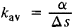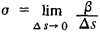# curvature

(redirected from Curvature (plane curve))
Also found in: Dictionary, Thesaurus, Medical.

## curvature

1. any normal or abnormal curving of a bodily part
2. Geometry the change in inclination of a tangent to a curve over unit length of arc. For a circle or sphere it is the reciprocal of the radius
Collins Discovery Encyclopedia, 1st edition © HarperCollins Publishers 2005

## curvature

(ker -vă-cher) See radius of curvature.
Collins Dictionary of Astronomy © Market House Books Ltd, 2006
The following article is from The Great Soviet Encyclopedia (1979). It might be outdated or ideologically biased.

## Curvature

(mathematics), a quantity characterizing the deviation of a curve or surface from a line or plane. The deviation of the arc MN (see Figure 1) of the curve L from the tangent MP at the point M may be characterized by the average curvature Kav of this arc, which is equal to the quotient of the angle a between the tangents at the points M and N to the length Δs of the arc MN:The average curvature of the arc of a circle is equal to the reciprocal of the circle’s radius and thus gives an intuitive characterization of the degree of curvature of a circle: the arc’s curvature increases with decreasing radius.Figure 1

The limit of the average curvature as the point N approaches the point M, that is, as s → 0, is called the curvature k of the curve L at the point M:The quantity R, the reciprocal of the curvature, is usually called the radius of curvature of the curve L at M.

If the curve L is the graph of a function y =f(x), then the curvature k of this curve may be calculated from the formulaThe curvature k of the curve L is, generally speaking, a function of the arc length s, measured from a certain point M on the curve. If L1 and L2 are curves whose curvatures, as functions of their respective arc lengths, are the same, then L1 and L2 are congruent, that is, they may be superimposed by a motion. For this reason, the definition of the curvature of a plane curve as a function of the arc length is usually called the natural (intrinsic) equation of this curve.

In order to characterize the deviation of a space curve L from the plane, we introduce the concept of torsion. The torsion σ at the point M of the curve is defined as the limit of the ratio of the angle β between the osculating planes to the curve at the points M and N to the length Δs of the arc MN as N approaches M:The angle β is taken to be positive if the rotation of the osculating plane at N as N approaches M is counterclockwise when viewed from M. The curvature and torsion, specified as functions of the arc length, define the curve L to within its position in space.

The deviation of a surface from a plane may be measured as follows. All possible planes are drawn through the normal at a given point M on the surface. The sections of the surface by these planes are called normal sections, and the curvatures of the normal sections at M are called the normal curvatures of the surface at this point. The maximum and minimum of the normal curvatures at the specified point M are called the principal curvatures. If k1 and k2 are the principal curvatures, then the quantities K = k1k2 and H = ½(k1 + K2) are called, respectively, the total curvature (or Gaussian curvature) and the average curvature of the surface at the point M. These curvatures of the surface determine the normal curvatures and may therefore be viewed as measures of the deviation of the surface from the plane. In particular, if K = 0 and H = 0 at all points of the surface, then the surface is a plane.

The total curvature does not change under bending (that is, deformations of the surface that do not change the length of curves on the surface). If, for example, the total curvature is equal to zero at all points of the surface, then each sufficiently small piece of the surface is applicable to a plane. The total curvature without reference to the surrounding space constitutes the central concept of the intrinsic geometry of the surface. The average curvature is related to the external shape of the surface.

The concept of curvature may be generalized to objects of a more general nature. For example, the concept of curvature arises in Riemann spaces and is a measure of the deviation of these spaces from Euclidean spaces.

### REFERENCES

Blaschke, V. Differentsial’naia geometriia i geometricheskie osnovy teorii otnositel’nosti Einshteina, vol. 1. Moscow-Leningrad, 1935. (Translated from German.)
Rashevskii, P. K. Kurs differentsial’noigeometrii, 4th ed. Moscow, 1956.
Pogorelov, A. V. Differentsial’naia geometriia, 5th ed. Moscow, 1969.

E. G. POZNIAK

## Curvature

a scarcely noticeable entasis, or convex swelling, given certain parts of a building. Curvature lends considerable sculptural expressiveness to a building and eliminates optical distortion when straight-sided forms of a building are viewed from a distance or when appreciable foreshortening occurs. It is found primarily in architecture employing the classical orders.